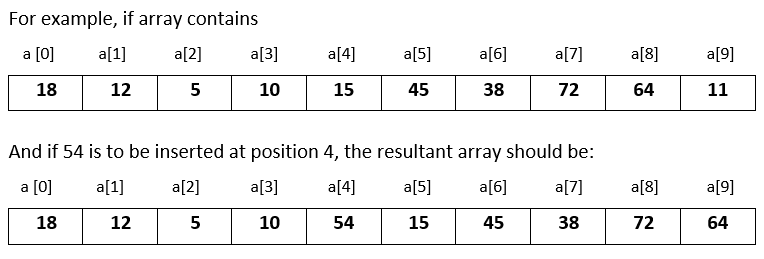# Input 10 numbers in 1d array and insert a number at the given position in C++

###### One Dimensional Array - Question 10

In this question, we will see how how to input 10 numbers in a one dimensional integer array and insert a number at a given position in the array in C++ programming. To know more about one dimensional array click on the one dimensional array lesson.

Q10) Write a program in C++ to input 10 numbers in a one dimensional integer array and input a number and a position. Now insert the number at that position by shifting the rest of the numbers to the right. The last element is therefore removed from the array.#### Program

``````#include <iostream>
#include <conio.h>

using namespace std;

int main()
{
int a, i,n,p;

cout<<"Enter 10 numbers\n";
for(i=0; i<10; i++)
{
cin>>a[i];
}

cout<<"Enter number and its position to insert in the array\n";
cin>>n>>p;
for(i=9; i>p; i--)
{
a[i]=a[i-1];
}
a[i]=n;

cout<<"\nModified array after inserting the number\n";
for(i=0; i<10; i++)
{
cout<<a[i]<<" ";
}
return 0;
}``````

#### Output

```Enter 10 numbers
18
12
5
10
15
45
38
72
64
11
Enter number and its position to insert in the array
54
4

Modified array after inserting the number
18 12 5 10 54 15 45 38 72 64```